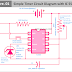Here, in this article, we are going to see the circuit diagram of a simple timer built with IC 555. We know the IC 555 is a timer IC that is easily available in the market and it is very easy to use also. So you can easily make a timer circuit by connecting a few electronic components with the IC.

First of all, let's see the circuit diagram.

## Circuit Components

• 9V DC Power Source or you can use a battery
• 1 Pcs 10K Resistor
• 1 Pcs NO Push Button Switch
• A 1 Mega Ohm Potentiometer or Preset
• A  16V, 1000 uF Capacitor
• An IC 555 Chip
• An LED to check the circuit output or you can use any other devices(such as Buzzer) or connect with other circuits.

## Connection Procedure

1. Connect pin 8 of the IC to the positive terminal of the power supply.
2. Connect pin 1 of the IC to the negative terminal of the power supply.
3. Connect one side terminal of the preset to the positive terminal of the power supply.
4. Short the middle terminal and another side terminal of the preset and connect them to pins 6 and 7 of the IC by shorting them.
5.  Connect the 1000 uF capacitor between the 6 no pin of the IC and the negative terminal of the power supply. Connect the negative terminal to the negative terminal of the power supply and the positive terminal to the 6 no pin of the IC.
6. Connect a 10K resistor between the positive terminal and negative terminal of the power supply.
7. Connect one terminal of the push button switch to pin no 2 of the IC and another terminal to the ground or negative terminal of the power supply.
8. Connect pin no 4 of the IC (Reset terminal) directly to the positive terminal of the power source.
9. Connect the positive terminal of the load(here is the LED) to the positive terminal of the power source and the negative terminal to pin no 3 of the IC(it is the output terminal).

## Working Principle of the Circuit

The time duration of this timer circuit can be achieved by setting the proper resistance value of the Preset. You can use this timer for up to 15-minute time duration.

Here is the formula to set the time duration by setting the resistance value.

T(in sec) = 1.1*R1*C1

Here, R1 is the value is to be set in the preset and C1 is the value of the capacitor(here we used 1000uF).

So if we want 1 minute or 60-second time duration then the value of the resistance is to be set in the preset as,

60 = 1.1*R1*1000

R1 = 60/(1.1*1000)

R1 = 55K

So we have to set the value to 55K in the preset. As, here we used a 1Mega ohm preset, so by placing the wiper at 55% position we can set the value to 55K. Or you can use a multimeter while setting the resistance value.

Now, you know how to find the resistance value for a particular time duration just set the value and get the timing. If you want a 10-minute time duration, just put 600 at the T(sec) in the formula.

Initially, when the circuit is turned on the LED will glow and it will be turned OFF or turned ON as per the time duration is set. Once the LED is glowing if we press the push button switch, it will start counting and the LED will be turned after the time duration as per set. For example, if we set 5 min, then the LED will turn off after 5 min.

A Simple Timer Circuit Diagram with IC 555Reviewed by Author on 7:00 am Rating: 5